International Journal of Electrical Components and Energy Conversion
Volume 1, Issue 2, June 2015, Pages: 55-62

Modelling Approach of Electric Cars Autonomy

Zaineb Gorbel, Yamina Chihaoui, Nader Barg, Mounir Yahyaoui, Souhir Tounsi

National School of Electronics and Telecommunications of Sfax, Sfax University, Sfax, Tunisia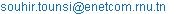(S. Tounsi)

Zaineb Gorbel, Yamina Chihaoui, Nader Barg, Mounir Yahyaoui, Souhir Tounsi. Modelling Approach of Electric Cars Autonomy. International Journal of Electrical Components and Energy Conversion. Vol. 1, No. 2, 2015, pp. 55-62. doi: 10.11648/j.ijecec.20150102.12

Abstract: The production of electric cars in large series is still hampered by their low autonomy due mainly to the low energy storage capacity of the batteries and the importance of overall losses of the power chain. Indeed, the electric vehicle monitoring strategy is a key factor in energy savings leading to improved of the autonomy. In this paper, we present a systemic control methodology of a synchronous motor with axial flux dedicated to electric traction, taking into account several constraints such as speed limit, the cost of the traction chain losses of the various components of the electric vehicle and the system reliability.

Keywords: Electric Cars, Autonomy, Scalar Control, Optimization, Modeling, Simulations.

1. Introduction

In this paper we present an approach to control all converter-engine designed by a systems design approach within the constraints of the application such as speed limit and interaction between the design and control in order to evaluate autonomy [1-5]. The model developed is implanted under the simulation environment Matlab / Simulink. The autonomy model takes into account the losses in the converter, the losses in the motor and energy recovery [1-10]. The highly parameterized model leads to a problem optimizing of the autonomy. In this context, this paper essentially has the following three parts:

Ÿ Presentation of the proposed control approach.

Ÿ Coupling of the autonomy model to the overall control model.

Ÿ Presentation and descriptions of the simulation results

2. Structure of the Power Chain

The structure of the power chain is with single engine to avoid skidding problem caused by the control of several motors at the same speed. The chosen type of static converter is with tow level supplyed by batteries DC bus voltage. The motor is a synchronous permanent magnet axial flux compact and with reduced production cost. The motor mechanical power transmitted to its shaft via a gear amplifier and a differential. The gear amplifier allows the car to reach the maximum speed and reduce the motor supply frequency, which leads to a considerable reduction of losses in the converter mainly dependent on the switching frequency also reduced losses in the motor leading to a significant improvement in autonomy [1-15].

3. Equation of Movement

The vehicle motion equation is derived from the fundamental relationship of dynamics [1-15]: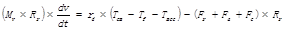(1)

Where Fr is the rolling resistance force, Fa is the aerodynamic force, Fc is the force of gravity, rd is the amplification ratio, Tem is the electromagnetic torque, Tf is the iron losses torque, Tmec is the mechanical losses torque, Rr is the radius of the wheel of the car, and v the speed of the car and Mv and the mass of the car.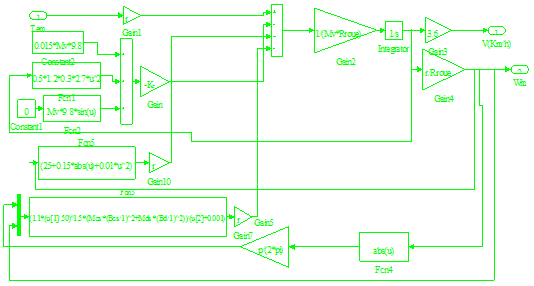Figure 1. Simulink model of the motion equation.

The vehicle motion equation is implanted under the environment Matlab / Simulink according to Figure 1.

4. Speed Control

The speed comparator outputs the amplitude of the reference currents minimizing the error between the reference speed and the response speed. Indeed, the reference speed is compared to the response speed. The comparator output drives a proportional / integral controller type (PI) to provide the amplitude reference currents minimizing the speed error. The Simulink model of the speed controller is shown in Figure 2 [3,4].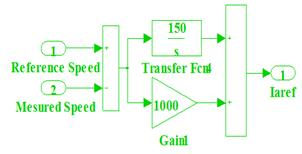Figure 2. Simulink Model of the speed regulator.

5. Current Regulation

Regulators currents allow the imposition of currents having the same shape and in phase with the back electromotive forces. Indeed, the reference currents are compared to the phases current of the motor. The outputs of the three comparators attack three regulators of the type proportional / integral (PI) to provide three reference voltages necessary to impose ideal currents in phase with the back electromotive forces and to minimize the error between the reference speed and response speed of the electric car [3,4].

Simulink model of the current regulator is illustrated in Figure 3: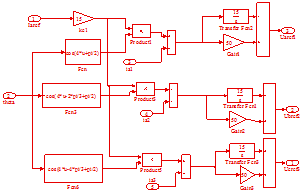Figure 3. Simulink model of the current regulator.

6. Model of the Back Electromotive Forces

The back electromotive forces are expressed by the three following equations [3,4]: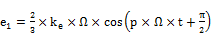(2)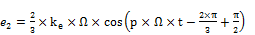(3)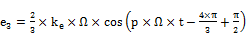(4)

Where ke is the electric constant of the motor and W is the angular velocity of the motor and p is the number of pole pairs.

These equations are implanted under the Matlab-Simulink environment according to Figure 4: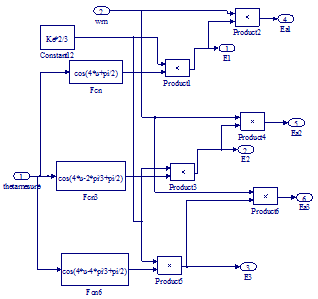Figure 4. Simulink model of the back electromotive forces.

7. Generator of Control Signals

The control signal generator compares the three reference voltages to a triangular signal having a frequency much greater than the voltages provided by the regulators of the currents. The output of each comparator drives a hysteresis variant between 0 and 1 to reproduce the control signals of the switches S1, S3 and S5. The speed controller and current controller adjusts the pulse width of the control signals so as to impose currents in phase with the back electromotive forces and minimize the error between the reference speed and the speed of response. The control signals of the switches S2, S4 and S6 are respectively complementary to the signals S1, S3 and S5 to avoid short circuits, the control pulses S2, S4 and S6 are shortened to avoid duplication between the two control signals of an arm. The Simulink model of the control signals generator is illustrated in Figure 5 [10-15]: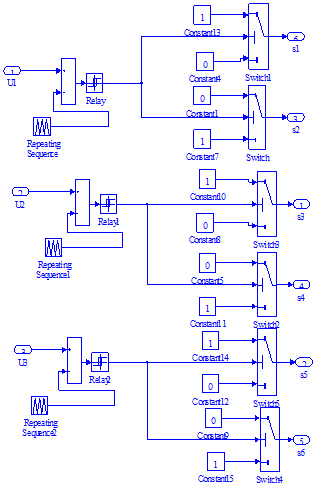Figure 5. Simulink Model control signal generator.

8. Model of the Motor-Converter

The motor is powered by a two-level voltage inverter in IGBT. Each phase of the motor is equivalent to a resistor in series with an inductance and a back electromotive force.

The three phases of the model is described by the following equations: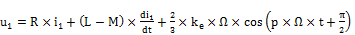(5)(6)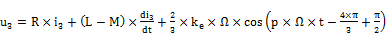(7)

Where R, L, M and ke are respectively the resistance, inductance, and mutual inductance and the electric motor constant, ii and ui are the current and the voltage of the phase i.

The electromagnetic torque is given by the following relationship:(8)

Where ei is the electromotive force against the i phase.

The model of the motor-converter is implanted under the Matlab-Simulink according to Figure 6:

9. Global Model of the Power Chain

The coupling of different models of the power of the electric car chain leads the global model implanted under the environment of Matlab / Simulink according to Figure 7:

10. Model of the Autonomy

The autonomy model (Au) takes into account of the recovered energy: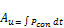(9)

With: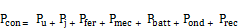(10)

Where Pcon is the power consumption, Pu is useful power, Pj are the copper losses, Pfer are the iron losses, Pmec are the mechanical losses, Pbatt are losses in the battery and Pond are the losses in the inverter.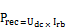(11)

Where Udc is the DC bus voltage and Irb is the recharge current of the batteries.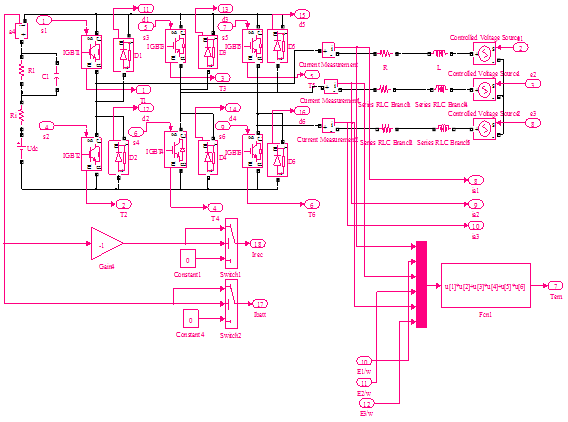Figure 6. Simulink Model of the motor-converter.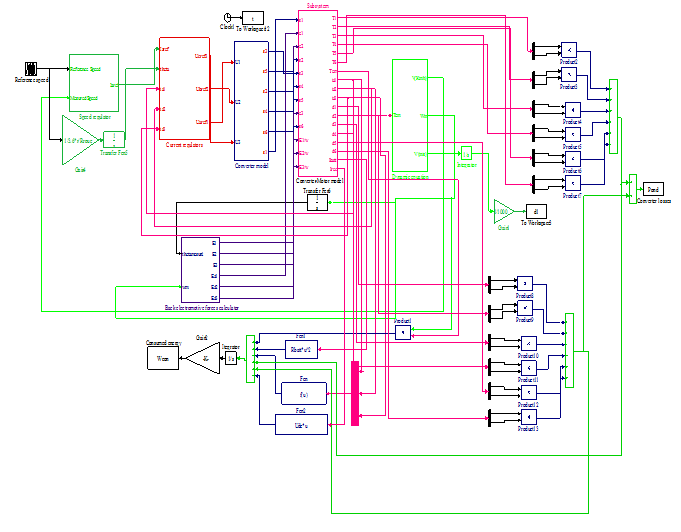Figure 7. Schematic model of Simulink block power chain.

The integral of the power consumed by the car at circulation mission illustrated in figure 8, gives the consummed energy.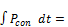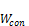(12)

Autonomy is calculated for repetitive cycle runs through the following relationship: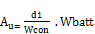(13)

Where d1 is the distance traveled by the car to speed cycle traveled by car and Wbatt is the total energy stored in the batteries.

11. Description of the Simulation Results

The reference speed relative to the car travels and the speed of response are shown in Figure 8:Figure 8. Speed Response.

Figure 8 shows that the response speed follows with great accuracy the reference speed, which shows the effectiveness of the chosen control technology.

Figure 9 shows the evolution of the electromagnetic torque: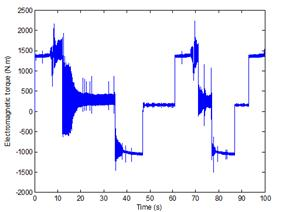Figure 9. Electromagnetic torque.

The electromagnetic torque has negative values since during generator operation phases, the engine torque is reversed.

Figure 10 shows the evolution of the phase current: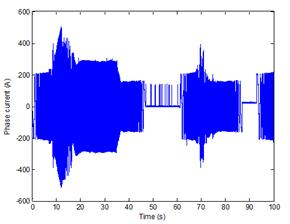Figure 10. Phase current.

This figure shows that the starting current is greatly reduced, leading to reduced energy consumption. This characteristic valid performance of the selected control technology.

Figure 11 shows the curves of the back electromotive force and phase current.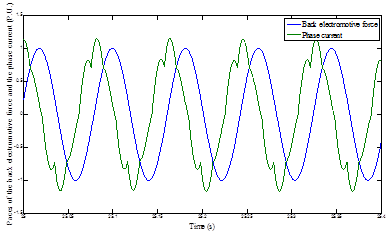Figure 11. Paces of the back electromotive force and phase current.

The figure 11 shows that there is a slight phase explained by the importance of the electric constant of the motor and low switching frequency.

Figure 12 illustrates the evolution of the phase voltage and the motor phase current.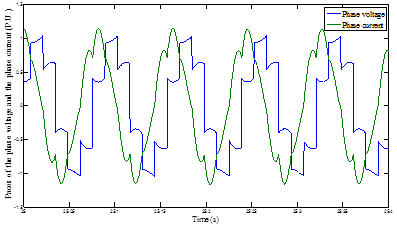Figure 12. Paces of the phase voltage and the phase current.

The figure 12 shows that the current waveform is close to a sinusoidal shape, confirming the effectiveness of the control technique chosen.

The slight voltage drop across the phase of the voltage of the bearing is essentially caused by the voltage drop across the IGBT transistors.

Figure 13 illustrates the evolution of the iron losses dissipated in the motor: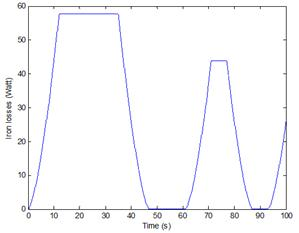Figure 13. Iron losses.

Figure 13 shows that the pace of the iron losses follows the curve of the speed since the iron losses are proportional to the frequency. These losses are small since the speed of the drive shaft is low (addition of a gear speed amplifier to reach the maximum speed).

Figure 14 illustrates the evolution of mechanical losses in the engine.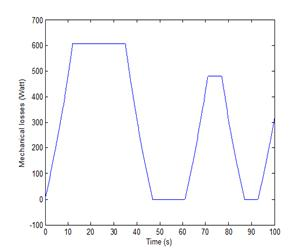Figure 14. Mechanical losses in the engine.

The mechanical losses in the motor evolve in a shape close to the shape of the car's speed cycle since they are proportional to the speed.

Figure 15 shows the evolution of copper losses in function of time.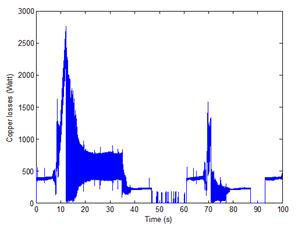Figure 15. Copper losses.

The copper losses show peaks during heavy acceleration phases for high stator currents. The average value of copper losses is reduced consideration the control technique imposes currents in phase with the back electromotive forces leading to a reduction of the current for a fixed power requirement. Thereafter, this reduction optimizes battery autonomy.

Figure 16 illustrates the evolution of the losses in the inverter in function of time.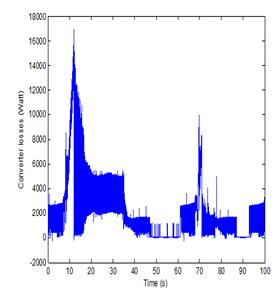Figure 16. Losses in the inverter.

The losses in the inverter show peaks during heavy acceleration phases for high stator currents. The average value of losses in the inverter is small view that the control technology imposes currents in phases with the back electromotive forces leading to a reduction current for a fixed power requirement.

Figure 17 illustrates the evolution of the energy consumed: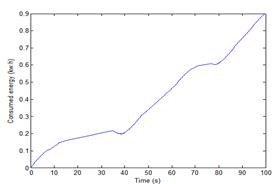Figure 17. Evolution of energy consumed.

Figure 17 shows the importance of reducing energy consumption which leads to increased autonomy.

Figure 18 shows the evolution of the recovered power.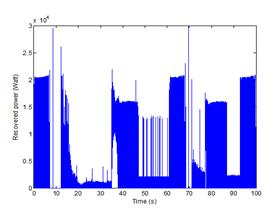Figure 18. Evolution of the recovered power.

Figure 18 shows the evolution of the recovered power that serves to increase the autonomy. The optimal value estimated by the model of autonomy is 93.3477 km.

12. Conclusion

In this paper, we presented a methodology for modeling of the autonomy of an electric car. This modeling is based on a control model reduced energy consumption and subsequently autonomy. This modeling approach is highly parameterized and leads to a problem of autonomy optimizing, determining factor for the production of electric cars in large series. This model is compatible to be solved by genetic algorithms method.

References

1. S. TOUNSI "Losses of the electromagnetic modeling and IGBT converters," International Int. J. Electric and Hybrid Vehicles (IJEHV), Vol. 5, No. 1, 2013, pp: 54-68 .
2. M. Hadj Kacem, S. and R. TOUNSI NEJI "Optimization of Cutting Frequency Electromagnetic Interuptor" International Journal of Computer Applications (0975 - 8887) Volume 67- No.21, April 2013, pp: 23-27.
3. S. TOUNSI "Comparative study of trapezoidal and sinusoidal control of electric vehicle power train," International Journal of Scientific & Technology Research (IJSTR), Vol. 1, Issue 10, November 2012.
4. M. Hadj Kacem, S. TOUNSI , R. NEJI "Systemic Design and Control of Electric Vehicles Power Chain," International Journal of Scientific & Technology Research (IJSTR), Vol. 1, Issue 10, November 2012.
5. M. Hadj Kacem, S. TOUNSI , R. NEJI "Control of an Actuator DC Energy-saving Dedicated to the Electric Traction," International Journal of Computer Applications: IJCA (0975 - 8887) Volume 54- No.10, pp. 20-25 September 2012.
6. S. TOUNSI " Control of the Electric Vehicles Power Chain with Electromagnetic Switches Reducing the Energy Consumption, "Journal of Electromagnetic Analysis and Applications (JEMAA) Vol.3 No.12, December 2011.
7. S. TOUNSI Mr. Hadj Kacem and R. NEJI " Design of Static Converter for Electric Traction "International Review on Modelling and Similations (IREMOS) Volume 3, N. 6, December 2010, pp. 1189-1195.
8. S. TOUNSI and R. NEJI: "Design of an Axial Flux Brushless DC Motor with Concentrated Winding for Electric Vehicles", Journal of Electrical Engineering (JEE), Volume 10, 2010 - Edition: 2, pp. 134-146.
9. S. TOUNSI , R. NEJI, and F. SELLAMI "Design Methodology of Permanent Magnet Motors Improving Performance of Electric Vehicles", International Journal of Modelling and Simulation (IJMS), Volume 29, No. 1, 2009.
10. M. CHAIEB, S. TOUNSI , R. and F. NEJI SELLAMI: "Design and optimization of axial permanent magnet machines for electric vehicle", Journal of Electrical Systems (JES) Volume 5, issue1, 2009, pp. 17-31.
11. N. CHAKER, I. Ben Salah, S. TOUNSI and R. NEJI: "Design of Axial-Flow for Motor Drive Application", J. Electromagnetic Analysis & Applications (JEMAA), 2009, 2, pp. 73-83.
12. MAFAKHFAKH Mr. HADJ KASEM, S. TOUNSI and R. NEJI "Thermal Analysis of Permanent Magnet Synchronous Motor for Electric Vehicle", Journal of Asian Electric Vehicles, Volume 6, Number 2, December 2008, pp. 1145-1151.
13. B. Ben Salah, A. MOALLA, S. TOUNSI , R. and F. NEJI SELLAMI "Analytic Design of a Permanent Magnet Synchronous Motor Dedicated to EV Traction With A Wide Range of Speed Operation", International Review of Electrical Engineering (IREE), Volume 3, No. 1 January - February 2008.
14. A. MOALLA, S. TOUNSI and R. NEJI "Determination of axial flux electric motor parameters by the analytic-finite Elements method", Journal of Electrical Systems, Volume 4, Issue 4, 2008, pp. 398-409.
15. M. CHAIEB, S. TOUNSI , R. and F. NEJI SELLAMI: "Design of a high-torque engine with two integrated motors axes Reducing the consumption electric vehicle", Journal of Electrical Systems (JES), volume 4, issue 1, 2008, pp. 1-23.

 Contents 1. 2. 3. 4. 5. 6. 7. 8. 9. 10. 11. 12.
Article ToolsAbstractPDF(362K)